# Sine and Cosine Functions

## Sine

The function y=sin(x) is periodic with period 2 π and the value of y is limited to the range [-1 1]. Thus, an ANN can be used in mapping configuration to compute the value of y=sin(x).

 Tip The number of training cases is very important when training an ANN. Specifically, the number of training cases limits the number of neurons in the hidden layer. In some few problems, it is possible to modify the number of training cases; in other problems the number of cases in the data set is given and cannot be changed making impossible to increase the number of neurons in the hidden layer without producing overfitting.

 Problem 1 Create a New Project called MappingSinCos using Neural Lab to build an appropriate training set to learn the functions: sin(x) and cos(x) as shown in the figure (we will use a General Purpose network application of a Multi-layer Network, you can save some typing by checking the Performace.lab checkbox in the project files). The training set will have 512 training cases using uniformly distributed values for x in the range from 0 to 2π.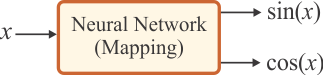Solution 1 Edit the BuildTrainSet.lab file and then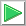Run click the button to execute the code. If you do not have any errors, the training set will be generated and displayed on the variable list and the file list.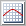Graph click the button to open the graph viewer and review the training set. Select the variables as shown.

 MappingSinCos\BuildTrainSet.lab int numCases = 512; //_________________________________________ Build the input Vector x; x.CreateSeries(0.0, 2.0*3.1415926, numCases); // 0, ..., 6.28 Matrix trainSetInput; trainSetInput = x; //_________________________________________ Save the input trainSetInput.Save(); //_________________________________________ Build the target Vector sinx = sin(x); Vector cosx = cos(x); Matrix trainSetTarget = sinx; trainSetTarget.AppendRight(cosx); //_________________________________________ Save the target trainSetTarget.Save();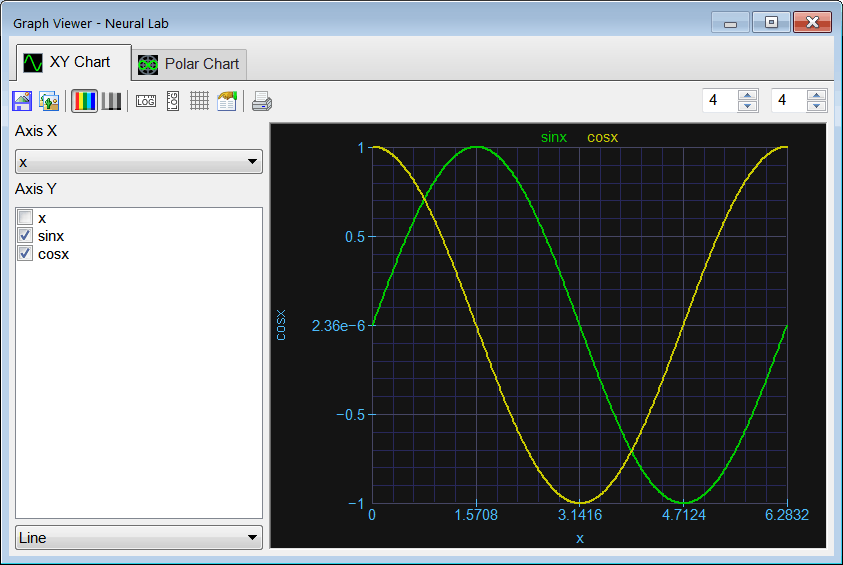Hint An easy way to explore a data set is by making click on the data set file as follows: Double click the file trainSetTarget.csv.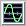XY Plot click the button to plot the data.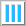See Columns click the button to plot each column. Move the scrollbar at the right to review each column plot. In this case, the first column (column 0) is a sine function and the second one (column 1) is a cosine function, see figure below.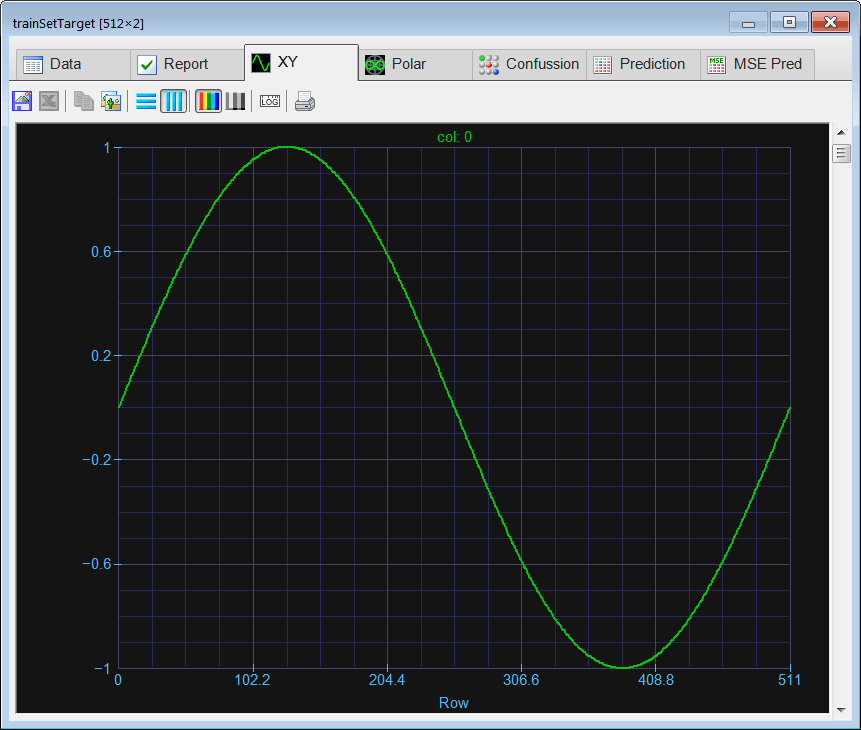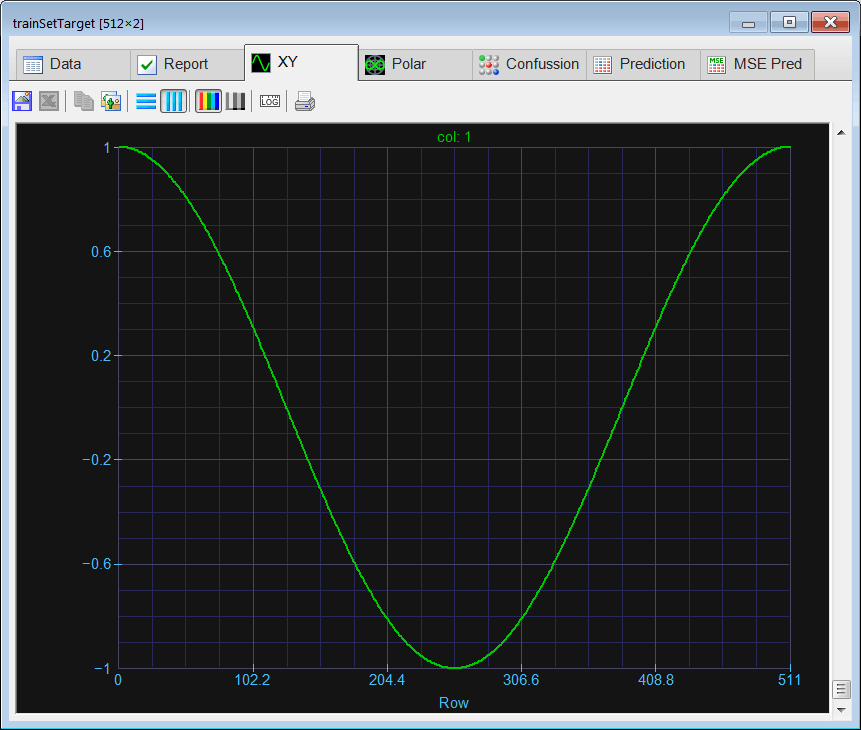Problem 2 Edit the BuildValidSet.lab file to build a validation set for the functions sin(x) and cos(x) using random values of x from 0 to 2π. Use 128 validation cases.

 Solution 2 After editing the file,Run click the button to execute the code. If you do not have any errors, the validation set will be generated and displayed on the variable list and the file list.Graph click the button to open the graph viewer and review the validation set. Select the variables as shown.

 MappingSinCos\BuildValidSet.lab int numCases = 128; //_____________________________________________ Build the input Vector x; x.CreateRandom(numCases, 0.0, 2*3.1415926); Matrix validSetInput; validSetInput = x; //_____________________________________________ Save the input validSetInput.Save(); //_____________________________________________ Build the target Vector sinx = sin(x); Vector cosx = cos(x); Matrix validSetTarget = sinx; validSetTarget.AppendRight(cosx); //_____________________________________________ Save the target validSetTarget.Save();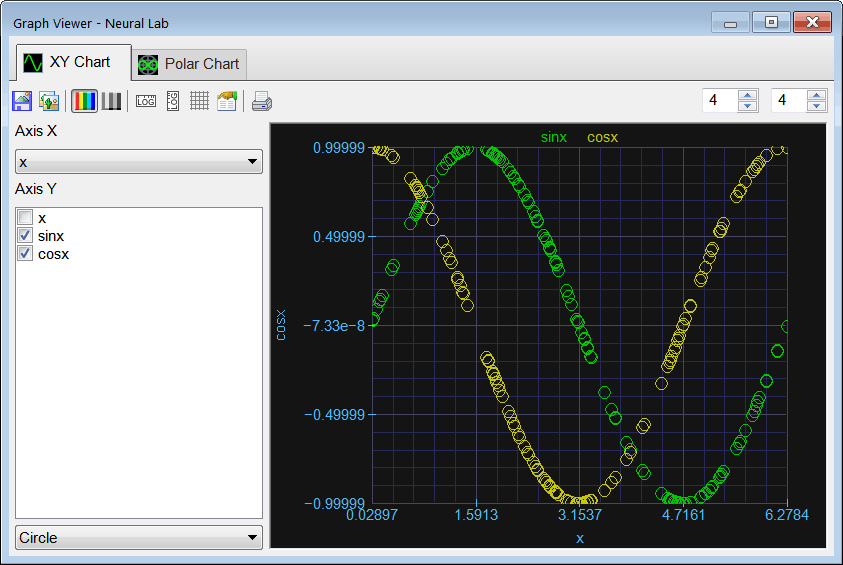Tip Even though it is possible to use only one training method to train a network, it is also possible and more reliable to use a combination of two (or more) methods. The training must start with a non-greedy method such as: Simulated Annealing or Genetic Algorithm. Next, the training can be improved using: the conjugate gradient method (TrainConjGrad), the Levenberg Marquardt method (TrainLevenMar), or the variable metric method (TrainVarMetric) as shown in the figure. Any of the improving training methods take two parameters: the number of epochs and the desired goal.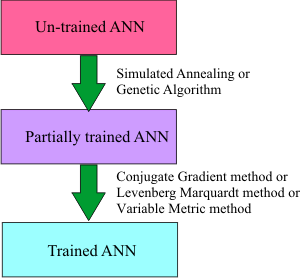Tip The training process may include any training method. In most cases the training process is limited by time, computing resources or human patient. For instance the training described in the figure below begins by using simulated annealing, and then it continues using a genetic algorithm and ends with the conjugate gradient method.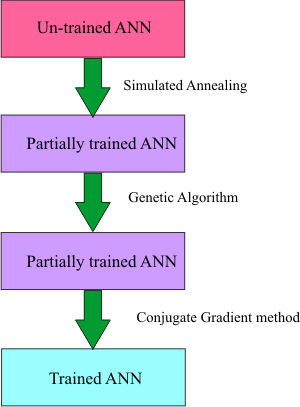Tip The method of Levenberg Marquardt requires great amount of memory, and it can only be used when the network has very few outputs. For those applications that require a network with many outputs the method of conjugate gradient or variable metric should be used.

 Tip All the training methods have a set of parameters that need to be adjusted. Generally speaking, if the number of iterations or number or epochs increases, the running time increases. Always train an ANN using the maximum number of iterations as the time allows. A high number of iterations bring the possibility of a good training.

 Problem 3 An ANN was trained using simulated annealing with 100 iterations per temperature. When the number of iterations was increased to 200, the training did not improve. What conclusions can be obtained from the experiment?

 Problem 4 Use the Internet to find about the method of simulated annealing (cooling schedules, the metropolis algorithm, initial temperature, final temperature, etc.). Write a page about this method. You may include figures and graphs.

 Problem 5 An ANN was trained using simulated annealing with 100 iterations per temperature and 10 temperatures. When the number of temperatures was increased to 50, the training improved considerably. What conclusions can be obtained from the experiment? What actions should be taken?

 Problem 6 Use the Internet to find about genetic algorithms (initial population, probability of crossover, probability of mutations, etc.). Write a page about this method. You may include figures and graphs.

 Problem 7 An ANN was trained using a genetic algorithm with 10 generations on a population size of 100. When the number of generations was increased to 50, the training improved considerably. What conclusions can be obtained from the experiment? What actions should be taken?

 Problem 8 An ANN was trained using a genetic algorithm with 10 generations on a population size of 100. When the population size was increased to 200, the training improved insignificantly. What conclusions can be obtained from the experiment? What actions should be taken?

 Problem 9 Use the Internet to find a typical value for the probability of mutation and the probability of crossover for genetic algorithms.

 Problem 10 Edit the Train.lab file to design and train an ANN for the functions sin(x) and cos(x). Use one hidden layer and five neurons in this layer. As the number of outputs of this network is only one, the method of Levenberg Marquardt can be used. Train the ANN using simulated annealing, and the method of Levenberg Marquardt as shown: Simulated Annealing:      Number of iterations = 100      Number of Temperatures = 100      Initial Temperature = 15      Final Temperature = 0.01      Cooling Schedule = Linear      Number of Cycles = 4      Goal (desired mse) = 1.0e-12Levenberg Marquardt:      Number of Epochs = 1000      Goal (desired mse) = 1.0e-12Solution 10 After editing the file,Run click the button to execute the code. If you do not have any errors, the ANN will be trained when the code execution stops. Double click the network in the variable list to review the network. Then, select inputTrainSet.csv file and the targetTrainSet.csv file. You will be able to review the network behavior in real time by moving the scrollbar at the right. You can automatically generate the network code by using the Generate network code from the toolbar.

 MappingSinCos\Train.lab //_________________________ Network Setup LayerNet net; net.Create(1, 12, 0, 2); net.SetInScaler(0, 0.0, 2.0*3.1415927); // Input 0: values are from 0.0 to 6.2833 net.SetOutScaler(0, -1.0, 1.0); // Output 0: values are from -1.0 to 1.0 net.SetOutScaler(1, -1.0, 1.0); // Output 1: values are from -1.0 to 1.0 //________________________ Load and set the training set Matrix trainSetInput; trainSetInput.Load(); Matrix trainSetTarget; trainSetTarget.Load(); net.SetTrainSet(trainSetInput, trainSetTarget, false); //________________________ Train using Simulated Annealing //net.TrainSimAnneal( //     100, //Number of Temperatures //     100, //Number Iterations //     15, //Initial Temperature //     0.001, //Final Temperature //     true, // Is Cooling Schedule Linear? //     4, // Number of Cycles //     1.0e-12 // Goal (desired mse) //); //________________________ Train using Genetic Algorithms net.TrainGenetic(      400, // Population Size      200, //Number Generations      1.5, // Over Population      0.001, // Probability of Mutation      0.8, // Probability of Crossover      1.0e-12); // Goal (desired mse) //________________________ Train using Levenberg Marquardt net.TrainLevenMar(1000,1.0e-12); // Epochs and Goal //net.TrainConjGrad(1000, 1.0e-12); //_____________________________ Save the trained network net.Save();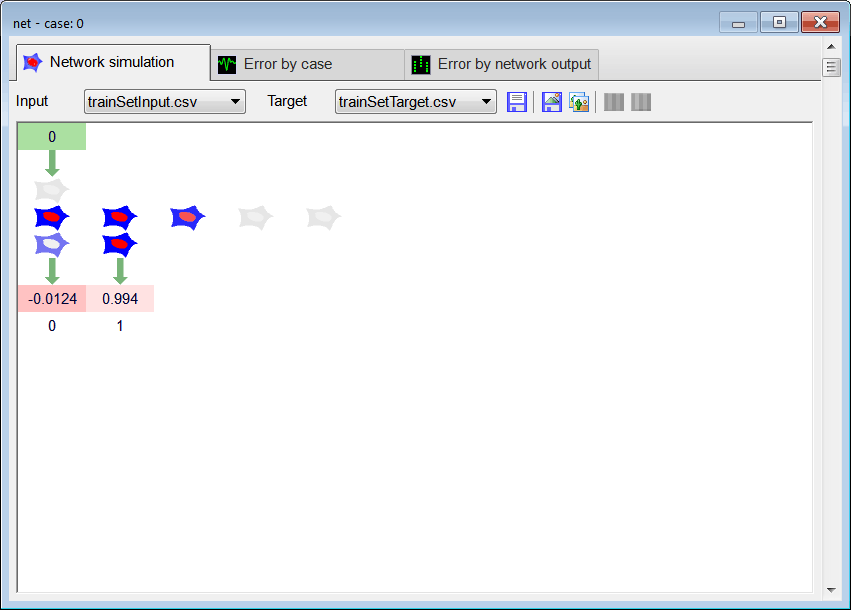Tip When looking at the network simulation, some of the output values are displayed in red. The red color indicates that the actual output network and the desired target are not equal for the current training case. You may use the scrollbar on the right side to review and analyze other training cases. When the network and the training are properly performed, the red color should appear in only very few cases or should not appear at all.

 Problem 11 Double click the network in the variable list of the last problem to see the network. Then, select inputTrainSet.csv file and the targetTrainSet.csv file.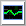Error by case click the tab to see the error by each training case.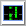Error by network output click the button to see the error for each output. What conclusions can be reached from these graphs?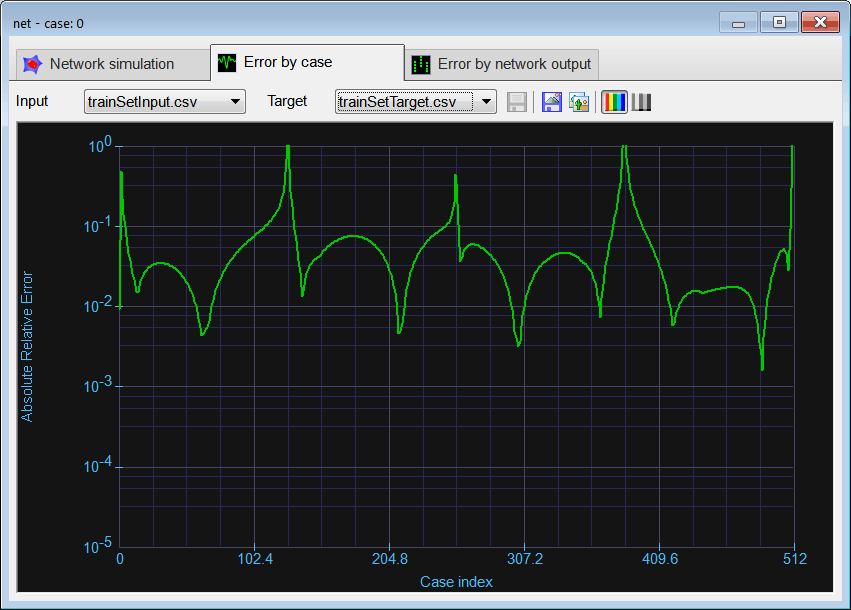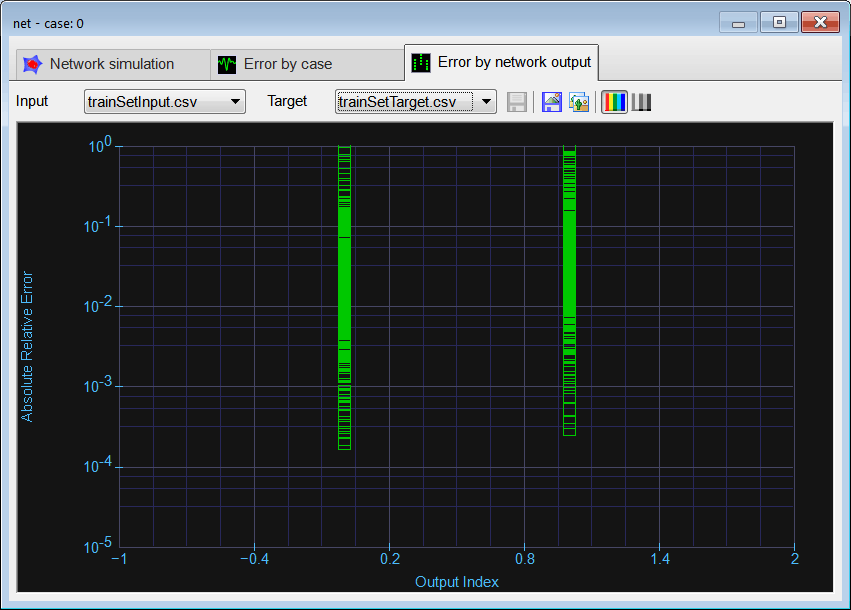Problem 12 In the network of problem 10, modify the number of hidden neurons to 10. Train the network and compute the error for each training case. What conclusions can be reached by comparing the error when the network had 5 neurons in the hidden layer?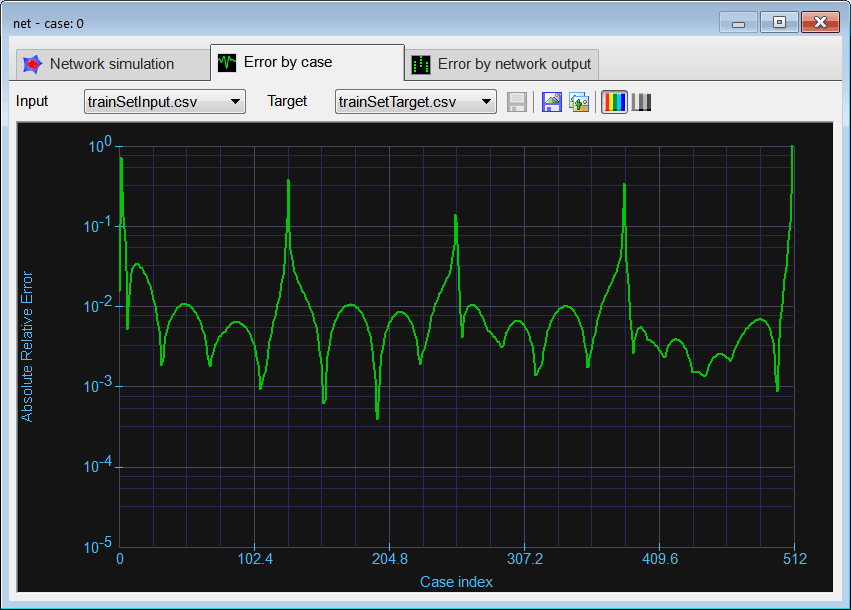Problem 13 In the network of problem 10, modify the number of hidden neurons to 20. Train the network and compute the error for each training case. What conclusions can be reached by comparing the error when the network had 5 and 10 neurons in the hidden layer?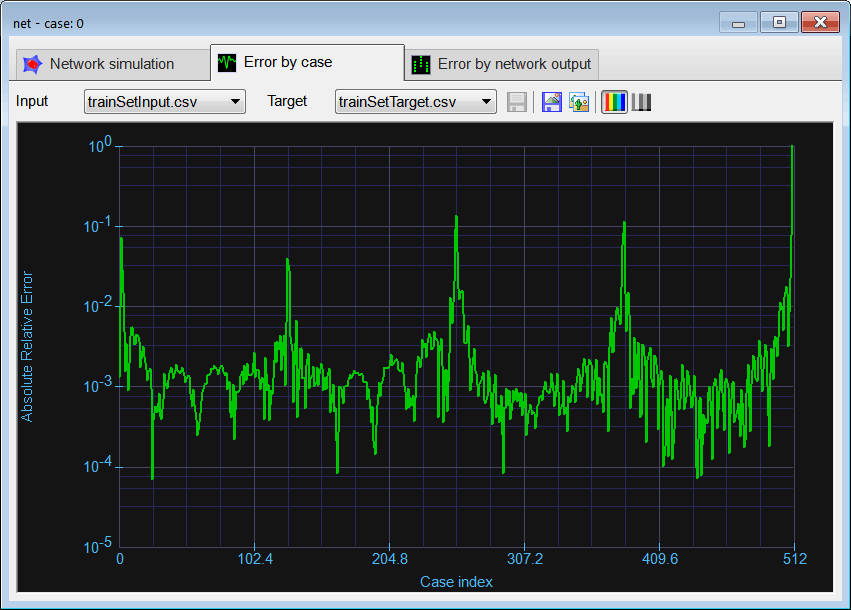Problem 14 Using the network trained in the last problem, double click the network to perform a simulation in real time use validSetInput.csv instead of trainSetInput.csv, and validSetTarget.csv instead of trainSetTarget.csv to perform the simulation. Is the behavior of the ANN different when the number of neurons in the hidden layer is modified?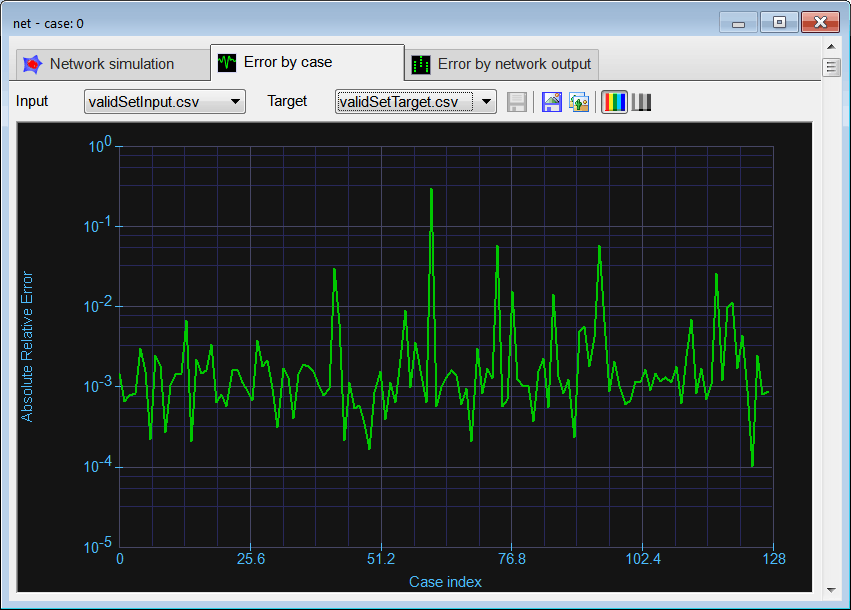Problem 15 Edit and run the CheckTraining.lab file to check the training: (a) Compute the mean squared error for the ANN (20 neurons in the hidden layer) using the training set. (b) Plot the error for each training case. (c) Save the plot as a vector image (checkTraining.emf and checkTraining.pdf)

 Problem 16 Edit and run the Validation.lab file to perform the validation of the ANN. (a) Compute the mean squared error for the ANN (20 neurons in the hidden layer) using the validation set. (b) Plot the error for each validation case. (c) Save the plot as a vector image (validation.emf and validation.pdf)

 Problem 17 Write some code to graph the mse for the validation and for training as a function of the number neurons in the hidden layer from 1 to 20. This test may last several hours.

 Solution 17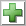Add File click the button to create a new code file called PerformanceTest. Write the code show below.Run click the button to execute the code. You will be asked to save the file, set the file name to PerformanceTest.lab. If you do not have any errors, the mse for both training and validation will be displayed in the variable list.Graph click the button to open the Graph Viewer and select the variables as shown. Be sure to use a logarithmic scale on the Y-axis. What conclusions can be obtained from the graphs?

 MappingSinCos\PerformanceTest.lab //________________________ Load and set the training set Matrix trainSetInput; trainSetInput.Load(); Matrix trainSetTarget; trainSetTarget.Load(); //_______________________ Load the validation set Matrix validSetInput; validSetInput.Load(); Matrix validSetTarget; validSetTarget.Load(); //________________________ Network setup int numHidden = 1; LayerNet net; Matrix output; Vector mseTraining; mseTraining.Create(20); Vector mseValidation; mseValidation.Create(20); for(numHidden = 12; numHidden<20; numHidden++) {      net.Create(1, numHidden, 0, 2);      net.SetInScaler(0, 0.0, 2.0*3.151927);      net.SetOutScaler(0, -1.0, 1.0);      net.SetOutScaler(1, -1.0, 1.0);      net.SetTrainSet(trainSetInput, trainSetTarget, false);      //      net.TrainSimAnneal(100, 100, 15, 0.01, false, 4, 1.0e-12);      //net.TrainGenetic(100, 100, 1.5, 0.01, 0.7, 1.0e-12);      //net.TrainLevenMar(1000,1.0e-12);      //_____________________________________________ check training           output = net.Run(trainSetInput);      mseTraining[numHidden] = ComputeMse(output, trainSetTarget);      //_____________________________________________ validation           output = net.Run(validSetInput);      mseValidation[numHidden] = ComputeMse(output, validSetTarget); } mseTraining.Save(); mseValidation.Save();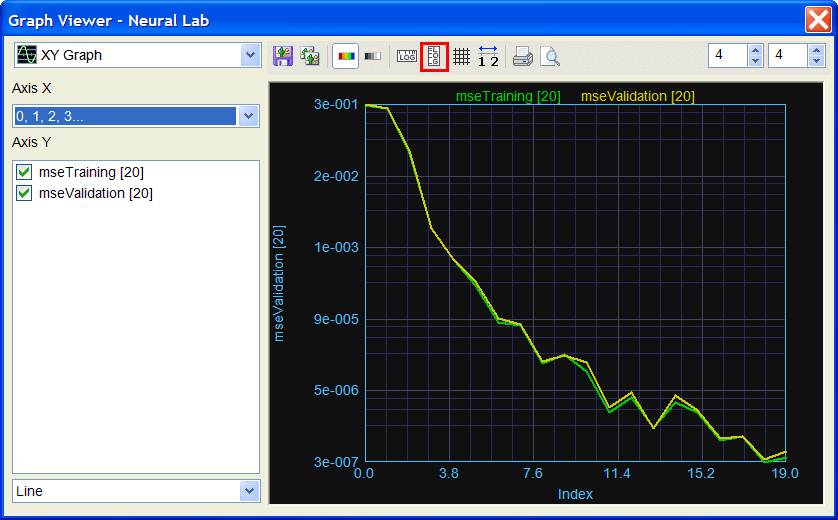Problem 18 Choose an appropriate number of neurons in the hidden layer and train the network. Write the file TrainSim.lab to compare the actual output of the network and the target using the training set.

 MappingSinCos\TrainSim.lab LayerNet net; net.Load(); //_____________________________________ Input Matrix trainSetInput; trainSetInput.Load(); //_____________________________________ Target Matrix trainSetTarget; trainSetTarget.Load(); Vector sinTarget = trainSetTarget.GetColVec(0); Vector cosTarget = trainSetTarget.GetColVec(1); //_____________________________________ ANN output Matrix output = net.Run(trainSetInput); Vector sinNN = output.GetColVec(0); Vector cosNN = output.GetColVec(1);

 Problem 19 From Neural Lab toolbar click on Learning Conflicts to analyze the training set. The figure below shows the learning conflicts in the first column in the training set. You can optionally, compute the learning conflicts in the second column in the training set. The Learning Conflicts tool allows you to find errors in your datasets.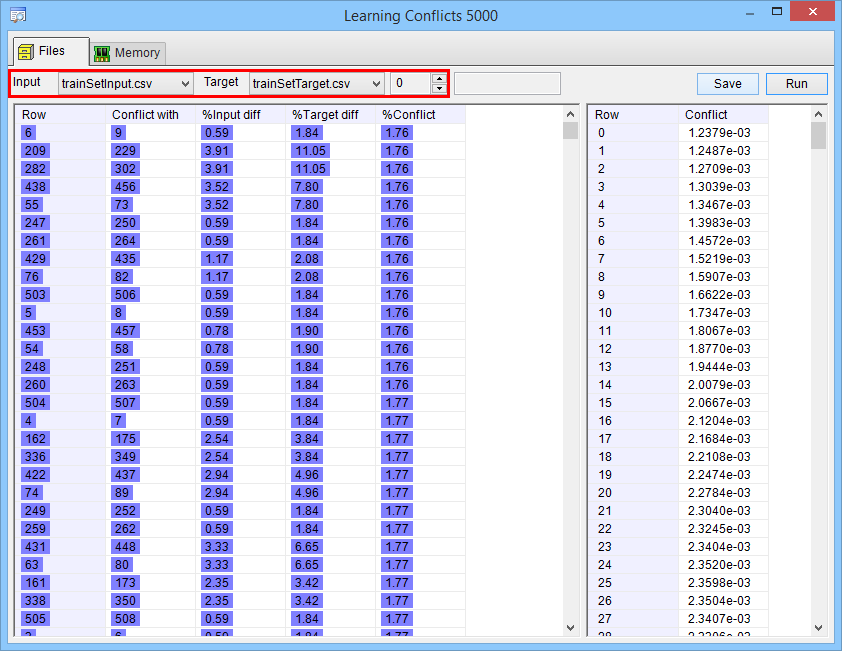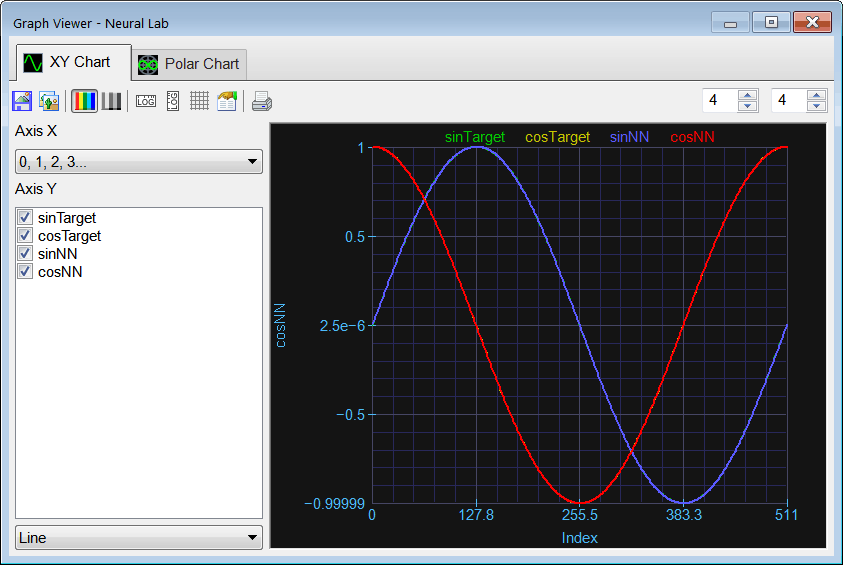Problem 20 Choose an appropriate number of neurons in the hidden layer and train the network. Write the file ValidSim.lab to compare the actual output of the network and the target using the validation set. As the validation set has random input points, we cannot use the original validation set. Instead we use a uniformly distributed validation set as shown (note that the number of points cannot be the same as the training set; in fact the number of points must be chosen carefully so that the cases in the validation set are the same as those in the training set).

 MappingSinCos\ValidSim.lab LayerNet net; net.Load(); int numCases = 300; //_____________________________________________ Build the input Vector x; x.CreateSeries(0.0, 2.0*3.1415926, numCases); // 0, ..., 6.28 //_____________________________________ Target Vector sinx = sin(x); Vector cosx = cos(x); //_____________________________________ ANN output Matrix output = net.Run(x); Vector sinNN = output.GetColVec(0); Vector cosNN = output.GetColVec(1);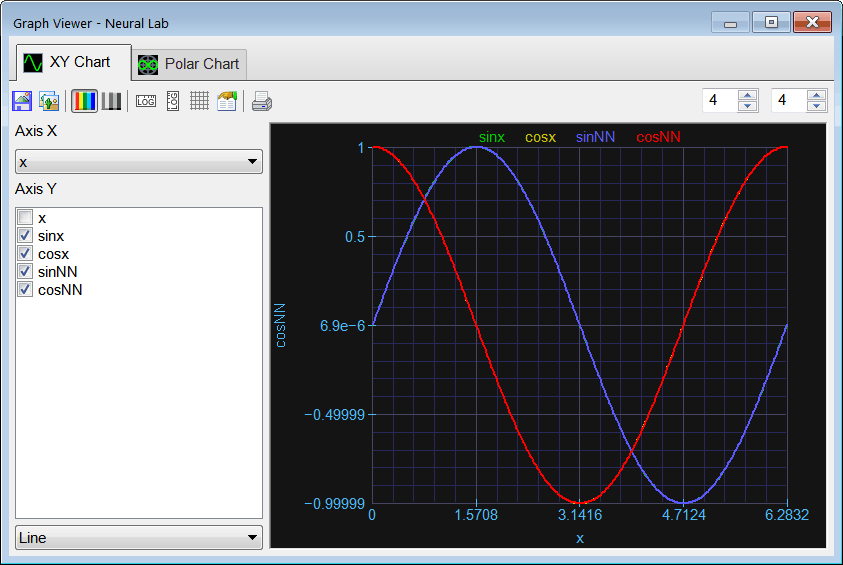Problem 21 Create an independent application for Microsoft Windows using C++ to compute the sine and cosine of a value using an ANN.

 Solution 21 For this problem you will require Microsoft Visual Studio and Wintempla. Links to download Microsoft Visual Studio and Wintempla are found in the main page of this tutorial (Academic SoftwareInstall Microsoft Visual Studio, if you have not done soInstall Wintempla, if you have not done soOpen Microsoft Visual Studio > File > New > Project > Visual C++ > Wintempla > Dialog Application (Set the name to Calculator and set an appropriate location to create the project)From Microsoft Visual Studio open Wintempla Tools>WintemplaDraw five labels, five text boxes and one button as shown. Be sure to assign the names as shown in the figure. Edit the file Calculator.h as shown below (write only what it is marked in red)Edit the file Calculator.cpp as shown below (write only what it is marked in red)Run the program Debug > Start debugging (if you have no errors, the calculator will open)Be sure the program can locate the net.lay file. Microsoft Visual Studio will try to open this file at the Calculator\Calculator folder. You need to copy the net.lay file from its original location to Calculator\Calculator\net.lay.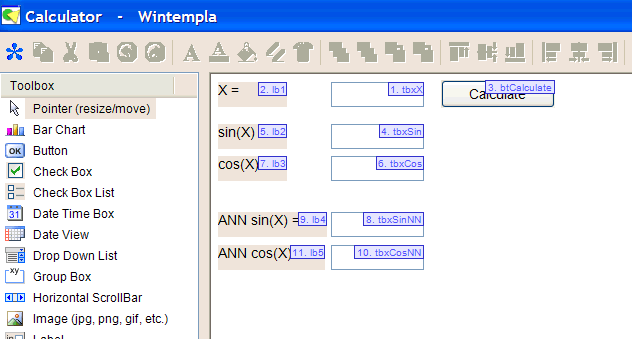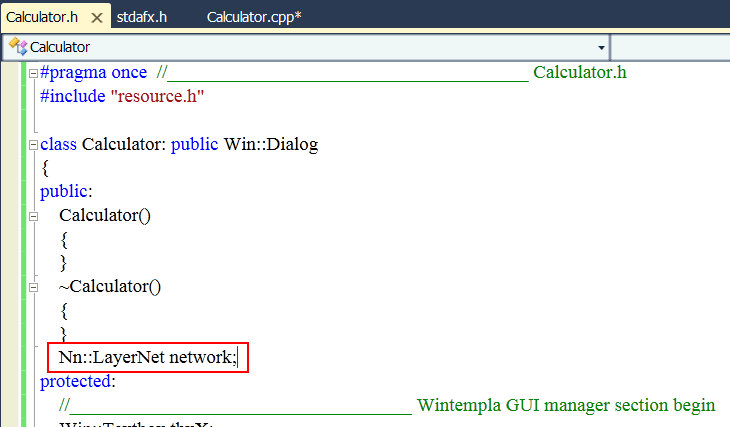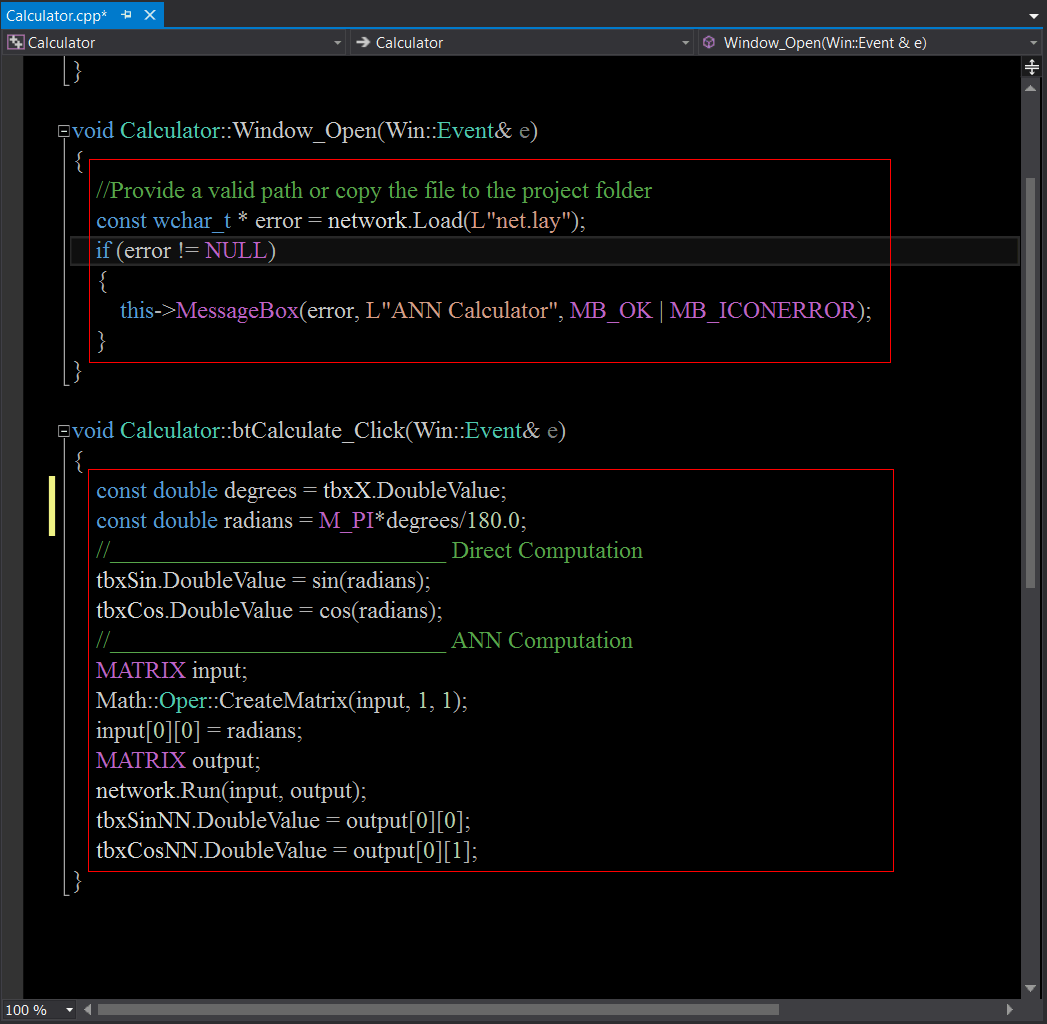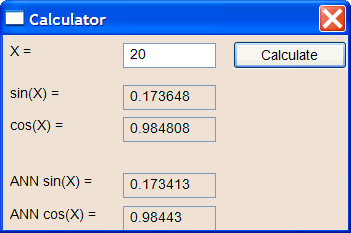Problem 22 Generate a report in Microsoft Word. Write some conclusions in the report focusing on the problems that were faced during the simulation and how these problems were or could be solved.